Courses

# Test: Newton’s First Law of Motion

## 10 Questions MCQ Test Physics Class 11 | Test: Newton’s First Law of Motion

Description
This mock test of Test: Newton’s First Law of Motion for JEE helps you for every JEE entrance exam. This contains 10 Multiple Choice Questions for JEE Test: Newton’s First Law of Motion (mcq) to study with solutions a complete question bank. The solved questions answers in this Test: Newton’s First Law of Motion quiz give you a good mix of easy questions and tough questions. JEE students definitely take this Test: Newton’s First Law of Motion exercise for a better result in the exam. You can find other Test: Newton’s First Law of Motion extra questions, long questions & short questions for JEE on EduRev as well by searching above.
QUESTION: 1

### If the net external force on the body is zero, its:

Solution:

Given, Fext = 0.
∵ F = ma
∴ a = 0 [as m cannot be 0]

QUESTION: 2

### When a bull pulls a cart, the force that helps the bull to move forward is the force exerted by:

Solution:

Bull exert the force on the ground, and ground exert equal force on cart and bull as well which help them to move forward.

QUESTION: 3

### Dust can be removed out of a blanket using:

Solution:

When a blanket is bitten with a stick or something then particles of blanket come into motion while dust particles remains in rest thus comes out which shows law of inertia.

QUESTION: 4

Which law governs the phenomenon that a tablecloth is pulled out from under a setting of chinaware without damaging it?

Solution:

According to Newton’s first Law, the cloth is set in motion but the chinaware continues to remain in its state of rest; and hence it is not damaged.
Hence, option A is correct.

QUESTION: 5

Two masses are in the ratio 1:5. What is ratio of their inertia?

Solution:

Force of inertia = ma

Let the masses be 1x and 5x

Force of inertia for 1st body= 1x * a

Force of inertia for 2nd = 5x * a

Ratio= x * a / 5x * a = 1:5

QUESTION: 6

We slip on a rainy day due to ______.

Solution:

On a rainy day, the rainwater forms a layer on the road.
This layer works like a lubricant which decreases the friction. Due to less friction, we slip on a rainy day.

QUESTION: 7

A 6 kg object is subject to three forces
F1 = 20+ 30j N
F2 = 8i - 50j N
F3 = 2i + 2j N
Find the acceleration of object.

Solution:

F = F1 + F2 + F3 = 30i - 18j
F = ma
Substituting F and m = 6 kg (given) in the above equation we get,
a = 5i - 3j
Hence (b) is correct choice

QUESTION: 8

Passengers in a bus lean forward as bus suddenly stops. This is due to

Solution:

When the bus moves, the passenger's body comes in the state of motion but when the bus stops the lower part of the body which is in contact with the floor comes in the state of rest whereas the upper part of the body still remains in the state of motion and because of this the upper part of the body falls in the forward direction.

This is due to the presence of inertia

QUESTION: 9

A particle of mass 'm' original at rest, is subjected to a force whose direction is constant but whose magnitude varies with according to the relation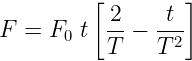,Where F0​ and T are constant.
Then speed of the particle after a time 2T is:

Solution:

Given:Speed of the particle after a time 2T,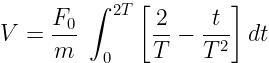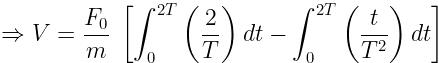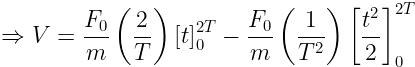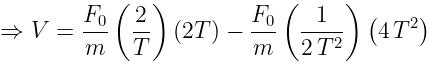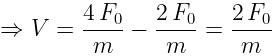QUESTION: 10

How is inertia used when riding a bicycle?

Solution:

Due to inertia, even if we stop paddling of motion, we will continue to move forward up to a certain distance as friction will finally make us stop.